# GSEB Solutions Class 6 Maths Chapter 1 Knowing Our Numbers InText Questions

Gujarat Board GSEB Textbook Solutions Class 6 Maths Chapter 1 Knowing Our Numbers InText Questions and Answers.

## Gujarat Board Textbook Solutions Class 6 Maths Chapter 1 Knowing Our Numbers InText Questions

Try These (Page 2)

Question 1.
Can you instantly find the greatest and the smallest numbers in each row?

(i) 382, 4972, 18, 59785, 750
59785 is the greatest and 18 is the smallest.

(ii) 1473, 89423, 100, 5000, 310

(iii) 1834, 75284, 111, 2333, 450

(iv) 2853, 7691, 9999, 12002, 124

Was that easy? Why was it easy?
Solution:
(ii) 89423 is the greatest and loo is the smallest.
(iii) 75284 is the greatest and ill is the smallest.
(iv) 12002 is the greatest and 124 is the smallest.
Yes, it was easy to identify the geatest or the smallest number in a row because a numeral containing more digits is greater.Try These (Page 2)

Question 1.
Find the greatest and the smallest numbers:
(a) 4536, 4892, 4370, 4452
(b) 15623, 15073, 15189, 15800
(c) 25286, 25245, 25270, 25210
(d) 6895, 23787, 24569, 24659
Solution:
(a) Each of the given numbers is having four digits and their digits at the thousands place are the same.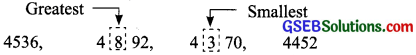On comparing the next digit of each number, we have 8> 5 > 4> 3.
The greatest number is 4892.
The smallest number is 4370.

(b) Each of the given numbers is having five digits and their two leftmost places have the same digits (i.e. 1 and 5).
On comparing the third leftmost digits, we have: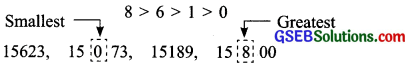The greatest number is 15800.
The smallest number is 15073.

(c) Each of the given numbers is having five digits and their three leftmost places have the same digits (i.e. 2, 5 and 2).
On comparing the fourth leftmost digits, we have: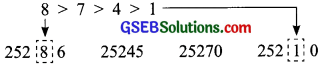The greatest number is 25286.
The smallest number is 25210.

(d) The given numbers are:The number 6895 is having four digits, so, it must be the smallest number. Each of the numbers 23787, 24569 and 24659 is having five digits and their leftmost digit is the same. On comparing the 2nd leftmost digit inAgain, the two leftmost places of 24569 and 24659 are having the same digits (i.e. 2 and 4).
On comparing their third leftmost digits, we have: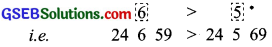The greatest number is 24659.
The smallest number is 6895.Try These (Page 3)

Question 1.
Use the given digits without repetition and make the greatest and smallest 4-digit numbers.
(a) 2, 8, 7, 4
(b) 9, 7, 4, 1
(c) 4, 7, 5, 0
(d) 1, 7, 6, 2
(e) 5, 4, 0, 3
Hint: 0754 is a 3-digit number.
Solution:
(a) The given digits are 2, 8, 7 and 4.
Ascending order of the given digits is: 2,4, 7, 8. .
The smallest 4-digit number = 2478.
The descending order of the given digits is: 8, 7, 4, 2…….
The greatest 4-digit number = 8742.

(b) Given digits are 9, 7, 4 and 1.
Ascending order of the given digits is: 1, 4, 7, 9….
The smallest 4-digit number = 1479.
Descending order of the given digits is: 9, 7, 4, 1…..
The greatest 4-digit number = 9741.

(c) Given digits are 4, 7, 5 and 0. Ascending
order of given digits is: 0, 4, 5, 7.
Keeping O in the 2nd leftmost place, the smallest number = 4057.
[The 0 in the is leftmost place is meaningless and 0457 becomes a 3-digit number]
Descending order of given digits is: 7, 5, 4, 0…..
The greatest 4-digit number = 7540.

(d) The given digits are 1, 7, 6 and 2.
Ascending order of given digits is: 1, 2, 6, 7.
The smallest 4-digit number 1267.
Descending order of given digits is: 7, 6, 2, 1.
The largest 4-digit number = 7621.

(e) Given digits are 5, 4, 0 and 3. Ascending
order of given digits is: 0, 3, 4, 5.
Keeping 0 in the 2nd leftmost place, the
smallest 4-digit number = 3045.
Descending order of given digits is: 5, 4, 3, 0.
The greatest 4-digit number 5430.Question 2.
Now make the greatest and the smallest
four-digit numbers by using any one digit twice.
(a) 3, 8, 7
(b) 9, 0, 5
(c) 0, 4,9
(d) 8,5, 1
Hint: Think in each case which digit you will use twice.
Solution:
(a) Given digits are 3, 8 and 7.
Ascending order of given digits is: 3, 7, 8.
The smallest 4-digit number = 3378.
(The smallest digit 3 is taken twice.)
Descending order of given digits is: 8, 7, 3.
The greatest 4-digit number = 8873.
(The greatest digit 8 is taken twice.)

(b) Given digits are 9, 0 and 5.
Ascending order is: 0, 5, 9.
Keeping 5 (instead of 0) at the leftmost place
and taking the smallest digit 0, twice, we have
the smallest 4-digit number = 5009.
Descending order is: 9, 5, 0.
The greatest 4-digit number 9950.
(The greatest digit 9 is repeated)

(c) Given digits are 0, 4 and 9.
Ascending order is: 0, 4, 9
The smallest 4-digit number = 4009.
Descending order is: 9, 4, 0
(Taking the smallest digit O, twice)
The greatest 4-digit number = 9940.
(Taking the greatest digit 9, twice)

(d) Given digits are 8, 5 and 1.
Ascending order is: 1, 5, 8
The smallest 4-digit number 1158.
(The smallest digit ¡ is taken twice.)
Descending order is: 8, 5, 1
The greatest 4-digit number = 8851.
(The greatest digit 8 is taken twice.)Question 3.
Make the greatest and the smallest 4-digit numbers using any four different digits with conditions as given.
(a) Digit 7 is always at ones place(Note, the number cannot begin with the

(b) Digit is always at tens place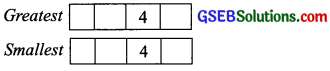(c) Digit 9 is always at hundreds place(d) Digit 1 is always at thousands placeSolution:
Digits in ascending order ate: 0, 1, 2, 3, 4, 5, 6, 7, 8 and 9; but 0 can never be taken at the leftmost place.
Digits in descending order are: 9, 8, 7, 6, 5, 4, 3, 2, 1 and 0.
(a) Keeping the digit ‘7’ at ones place, we have:The greatest 4-digit number = 9867.
The smallest 4-digit number = 1027.

(b) Keeping the digit 4 at tens place, we have: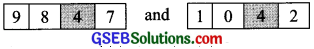The greatest 4-digit number = 9847.
The smallest 4-digit number = 1042.

(c) Keeping the digit 9 at the hundreds place, we have:The greatest 4-digit number = 8976.
The smallest 4-digit number = 1902.

(d) Keeping the digit I at the thousands place, we have: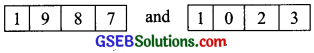The greatest 4-digit number = 1987.
The smallest 4-digit number = 1023.Question 4.
Take two digits, say 2 and 3. Make 4-digit numbers, using both the digits equal number of times.
Which is the greatest number?
Which is the smallest number?
How many different numbers can you make in all?
Solution:
The given digits are 2 and 3. For making 4-digit numbers, we will repeat the given digits equal number of times.
The possible 4-digit numbers are 3322, 2233, 2323, 3232, 3223 and 2332.
Among these, we have
(i) The greatest number = 3322.
(ii) The smallest number = 2233.
(iii) We can make six different 4-digit numbers.

Try These (Page 4)

Question 1.
Think of five more situations where you compare three or more quantities.
Solution:
Do it yourself.Try These (Page 5)

Question 1.
Arrange the following numbers in ascending order:
(a) 847, 9754, 820, 571
(b) 9801, 25751, 36501, 38802
Solution:
Arranging in ascending order means rewriting in increasing order.
(a) Given numbers are 847, 9754, 8320 and 571.
We write them in ascending order as: 571, 847, 8320, 9754.

(b) Given numbers are 9801, 25751, 36501 and 38802.
We write them in ascending order as: 9801, 25751, 36501, 38802.

Question 2.
Arrange the following numbers in descending order:
(a) 5000, 7500, 85400, 7861
(b) 1971, 45321, 88715, 92547
Make ten such examples of ascending/descending order and solve them.
Solution:
Arranging in descending order means rewriting in decreasing order.

(a) Given numbers are 5000, 7500, 85400 and 7861.
We write them in descending order as: 85400, 7861, 7500, 5000.

(b) Given numbers are 1971, 45321, 88715 and 92547.
We write them in descending order as: 92547, 88715, 45321, 1971.Try These (Page 6)

Question 1.
Read and expand the numbers wherever there are blanks.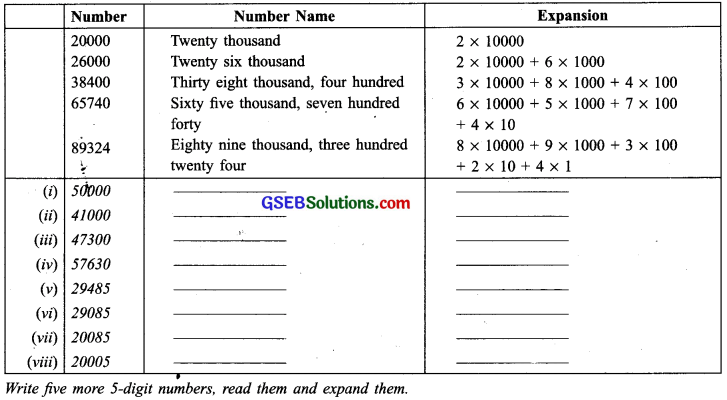Solution:Now, we consider the following 5 numbers:
(i) 90000
(ii) 27000
(iii) 36900
(iv) 36009
(v) 30069
Let us read and expand them:Note :
1. The greatest 1-digit number 9 and 9 + 1 = lo, the smallest 2-digit number.

2. The greatest 2-digit number = 99 and 99 + 1 = 100, the smallest 3-digit number.

3. The greatest 3-digit number = 999 and 999 + 1 1000, the smallest 4-digit number.

4. The greatest 4-digit number = 9999 and 9999 + 1 10000, the smallest 5-digit number.

5. Thus we conclude that:
[The greatest I-digit number] + 1 the smallest 2-digit number.
[The greatest 2-digit number] + 1 = the smallest 3-digit number.
[The greatest 3-digit number] + 1 = the smallest 4-digit number.
[The greatest 4-digit number] + 1 = the smallest 5-digit number.

6. The greatest 5 digit number = 99999 and 99999 + 1 = 100000. 100000 is the smallest 6-digit number and its number name is one lakh.
Also 100000 = 100 x 1000. i.e. one lakh = 100 thousands.

7. The smallest 7-digit number = 1000000 ten lakh.
The smallest 8-digit number 10000000 = one crore.
The smallest 9-digit number 100000000 = ten crore.

8. 1 hundred = 10 tens
1 thousand = 10 hundreds = 100 tens
1 lakh = 100 thousands 1000 hundreds
1 crore = 100 lakhs = 10000 thousandsTry These (Page 7)

Question 1.
Read and expand the numbers wherever there are blanks: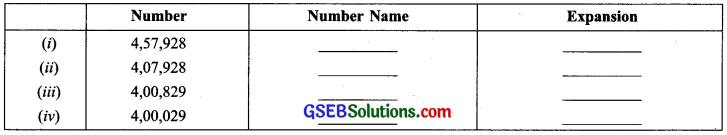Solution:Question 2.
Complete the following pattern:
9 + 1 = 10
99 + 1 = 100
999 + 1 = ……………
9,999 + 1 = ……………
99,999+1 = ……………
9,991999 + 1 = ……………
99,99,999 + 1 = 1,00,00,000
Solution:
9 + 1 = 10
99+1=100
999+1 =1,000
9,999 + 1 = 10,000
99,999 + 1 = 1,00,000
9,99,999 + 1 = 10,00,000
99,99,999 + 1 = 1,00,00,000Try These (Page 8)

Question 1.
1. What is 10 – 1 = ?
2. What is 100 – 1 = ?
3. What is 10,000 – 1 = ?
4. What is 1,00,000 – 1 = ?
5. What is 1,00,00,000 – 1 = ?
Hint: Use the above pattern.
Solution:
Using the pattern, we have:
10 – 1 = 9
loo – 1 = 99
1,000 – 1 = 999
10,000 – 1 = 9,999
1,00,000 – 1 = 99,999
10,00,000 – 1 = 9,99,999
1,00,00,000 – 1 = 99,99,999
Using this pattern, we can say:
1. 10 – 1 = 9
2. 100 – 1 = 99
3. 10,000 – 1 = 9,999
4. 1 ,00,000 – 1 = 99,999
5. 1,00,00,000 – 1 = 99,99,999.Try These (Page 8)

Question 1.
Give five examples where the number of things counted would be more than a 6-digit number
Solution:
The least 6-digit number is 100000 (one lakh).
Examples with things more than 1 lakh:
(i) The, number of stars seen in a clear dark night.
(ii) Number of cars in Delhi.
(iii) Number of children in a big city.
(iv) Number of grains in a sack full of grains.
(y) Number of pages of notebooks of all students in a town.

Question 2.
Starting from the greatest 6-digit number write the previous five numbers in descending order
Solution:
The greatest 6-digit number is 999999
1st previous number = 999999 – 1 = 999998
2nd previous number = 999999 – 2 999.997
3rd previous number = 999999 – 3 = 999996
4th previous number = 999999 – 4 = 999995
5th previous number = 999999 – 5 = 999994
Writing these numbers in descending order, we
have: 999998, 999997, 999996, 999995, 999994.Question 3.
Starting from the smallest 8-digit number write the next five numbers in ascending order and read them.
Solution:
The smallest 8-digit number = 10000000
1st next number is: 10000000 + 1 = 10000001
2nd next number is: 10000000 +2 = 10000002
3rd next number is: 10000000 + 3 = 10000003
4th next number is: 10000000 +4 = 10000004
5th next number is: 10000000 + 5 = 10000005
Writing these numbers in ascending order, we
have: 10000001, 10000002, 10000003, 10000004, 10000005.
10000001: one crore one
10000002: one crore two
10000003: one crore three
10000004: one crore four
10000005: one crore fiveTry These (Page 11)

Question 1.
Read these numbers. Write them using placement boxes and then write their expanded forms:
(i) 475320
(ii) 9847215
(iii) 97645310
(iv) 30458094
(a) Which is the smallest number?
(b) Which is the greatest number?
(c) Arrange these numbers in ascending and descending orders.
Solution:
(i) 475320We read it as: four lakh seventy five thousand three hundred twenty.
Expanded form: 475320 = 4 x 100000 + 7 x 10000 + 5 x 1000 + 3 x 100 + 2 x 10 + 0

(ii) 9847215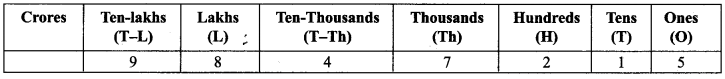We read it as: ninety eight lakh forty seven thousand two hundred fifteen.
Expanded form: 9847215 = 9 x 1000000 + 8 x 100000 + 4 x 10000 + 7 x 1000 + 2 x 100 + 1 x 10 + 5 x 1

(iii) 97645310We read it as: nine crore seventy six Iakh forty five thousand three hundred ten.
Expanded form: 97645310 = 9 x 10000000 + 7 x 1000000 + 6 x 100000 + 4 x 10000 + 5 x 1000 + 3 x 100 + 1 x 10 + 0

(iv) 30458094We read it as : three crore four lakh fifty eight thousand ninety four.
Expanded form : 304580943 x 10000000 + 4 x 100000 + 5 x 10000 + 8 x 1000 + 9 x 10 + 4 x 1
(a) The smallest number is 475320.
(b) The greatest number is 97645310.
(c) Ascending order 475320, 9847215, 30458094, 97645310,
Descending order: 97645310, 30458094, 9847215, 475320.Question 2.
(i) 527864
(ii) 95432
(iii) 18950049
(iv) 70002509
(a) Write these numbers using placement boxes and then using commas in Indian as well as International System of Numeration.
(b) Arrange these in ascending and descending orders.
Solution:
Reading these numbers, we have :
(i) 5,27,864: Five lakh twenty seven thousand eight hundred sixty four
(ii) 95,432: Ninety five thousand four hundred thirty two
(iii) 1,89,50,049: One crore eighty nine lakh fifty thousand forty nine
(iv) 7,00,02,509: Seven crore two thousand five hundred nine

(a) Using the placement boxes, we have:International System of Numeration
Using commas, we can re-write these numbers as:
(i) 5278M 5,27,8M ← (ludian System) = 527,864 ← (International System)
(ii) 95432 = 95,432 ← (Indian System) 95,432 ← (International System)
(iii) 18950049 = 1,89,50,049 ← (Indian System) = 18,950,049 ← (International System)
(iv) 70002509 = 7,00,02,509 ← (Indian System) = 70,002,509 ← (International System)
(b) Ascending order: 95,432; 5,27,864; 1,89,50,049; 7,00,02,509
Descending order: 7,00,02,509; 1,89,50,049; 5,27,864; 95,432Question 3.
Take three more groups of large numbers and do the exercise given above.
Solution:
Do it yourself.

Can you help me write the numeral (Page 11)

Question 1.
Write numerals for:
(a) Forty two lakh seventy thousand eight
(b) Two crore ninety lakh fifty five thousand eight hundred
(c) Seven crore sixty thousand fifty five
Solution: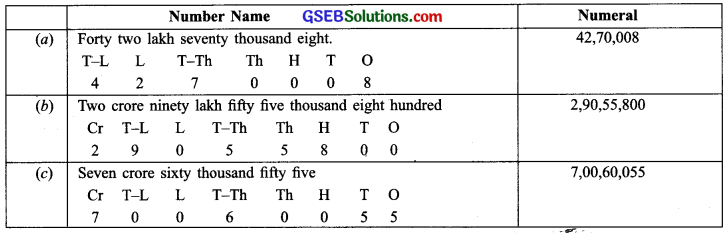Try These (Page 11)

Question 1.
You have the following digits 4, 5, 6, 0, 7 and 8. Using them, make five numbers each with 6 digits :
(a) Put commas for easy reading.
(b) Arrange them in ascending and descending order
Solution:
Five numbers each with 6 digits using the given digits are:
(i) 876540
(ii) 876450
(iii) 867540
(iv) 867405
(v) 876045

(a) Rewriting the above numbers using commas,
(i) 8,76,540
(ii) 8,76,450
(iii) 8,67,540
(iv) 8,67,405
(v) 8,76,045

(b) Ascending order: 8,67,405; 8,67,540; 8,76,045; 8,76,450; 8,76,540
Descending order: 8,76,540; 8,76,450; 8,76,045; 8,67,540; 8,67,405 .Question 2.
Take the digits 4, 5, 6, 7, 8 and 9. Make any three numbers each wiLlil digits. Put commas for easy reading.
Solution:
Three numbers each With- 8 digits using given digits are:
(i) 9,88,77,456
(ii) 9,88,77,465
(iii) 9,88,77,654

Question 3.
From the digits 3, 0 and 4, make five numbers each with 6 digits. Use commas.
Solution:
Five number each with 6 digits using the digits 3, 0 and 4 are:
(i) 5,44,330
(ii) 5,43,340
(iii) 5,40,340
(iv) 5,00,343
(v) 5,03,403

Try These (Page 12)

Question 1.
How many centimetres make a kilometre?
Solution:
1 km = 1000 m and 1 m = 100 cm
1 km = 1000 x loo cm = 1,00,000 cm
= one lakh cm
There are 1 lakh centimetres in a kilometre.Question 2.
Name five large cities in India? Find their population. Also, find the distance in kilometres between each pair of these cities.
Solution:
Do it yourself.

Try These (Page 13)

Question 1.
How many milligrams make one kilogram?
Solution:
1 kg = 1000 g and 1 g = 1000 mg
1 kg = 1000 x 1000 mg = 10,00,000 mg
= 10 lakh mg
There are ten-lakh milligrams in 1 kilogram.

Question 2.
A box contains 2,00,000 medicine tablets each weighing 20 mg. What is the total weight of all the tablets in the box in grams and in kilograms?
Solution:
Number of tablets = 2,00,000
Weight of one tablet = 20 mg
∴ Total weight of all tablets
= 20 x 200000 mg
= 4000000 mg
Since 1 kg 1000 g and 1 g 1000 mg
4000000 mg = $$\frac { 4000000 }{ 1000 }$$g = 4000 g
Thus, the total weight of all the tablets in grams
= 4000g.
Also, 4000000 mg = $$\frac { 4000000 }{ 1000×1000 }$$ = 4 kg
Thus, the total weight of all the tablets in
kilograms = 4 kgTry These (Page 13)

Question 1.
A bus started its journey and reached different places with a speed of 60 km/hour The journey is shown below.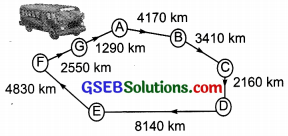(i) Find the total distance covered by the bus from A to D.
(ii) Find the total distance covered by the bus from D to G.
(iii) Find the total distance covered by the bus, If it starts from A and returns back to A.
(iv) Can you find the difference of distances from C to D and D to E?
(y) Find out the time taken by the bus to reach
(a) A to B
(b) C to D
(c) E to G
(d) Total journey
Solution:
(i) Distance covered by the bus for going from A to D
4170 km + 3410 km + 2160 km
= [4170 + 3410 + 2160] km = 9740 km

(ii) Total distance covered by the bus for going from D to G
= 8140 km + 4830 km + 2550 km
= [8140 + 4830 + 2550] km = 15520 km

(iii) Total distance covered by the bus for going round from A to A
= [Distance between A and D] + [Distance between D and G] + [Distance between G and A]
= [9740 km] + [15520 km] + [1290 km]
= [9740 + 15520 + 1290] km = 26550 km

(iv) [Distance between D and E] – [Distance between C and D]
= [8140 km] – [2160 km]
= [8140 – 2160] km = 5980 km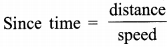And speed of the bus is 60 km/hour.
(a) Time taken by the bus to reach from A to B
= $$\frac { 4170km }{ 60km/hour }$$ = $$\frac { 4170 }{ 60 }$$ hour = 69 $$\frac { 1 }{ 2 }$$ hour

(b) Time taken by the bus to reach from C to D
= $$\frac { 2160 }{ 60 }$$ hours = 36 hours

(c) Time taken by the bus to reach from E to G
$$\frac { [4830km+2550 km] }{ 60 km/hour }$$ = $$\frac { 7380km }{ 60 km/hour }$$ = $$\frac { 7380 }{ 60 }$$
= 442 $$\frac { 1 }{ 2 }$$ hours

(d) Total time taken lÝy the bus for the total journey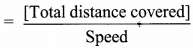$$\frac { 26550km }{ 60 km/hour }$$ = $$\frac { 26550 }{ 60 }$$hour
= 44 $$\frac { 1 }{ 2 }$$ hoursQuestion 2.
Raman’s Shop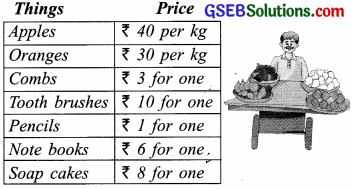The sales during the last year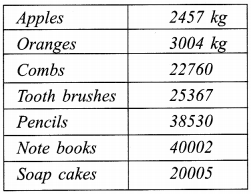(a) can you find the total weight of apples and oranges Raman sold last year?
Weight of apples = ………………. kg
Weight of oranges = ………………. kg
Therefore, total weight = ………………. kg
+ ………………. kg = ………………. kg
Answer – The total weight of oranges and
apples = ………………. kg.

(b) Can you find the total money Raman got by selling apples?

(c) Can you find total money Ramati got by selling apples and oranges together?

(d) Make a table showing how much money Raman received from selling each item.
Arrange the entries of amount of money received in descending order.
Find the item which brought him the highest amount.
How much is this amount?
Solution:
(a) Quantity of oranges and apples sold by Raman last year:
Weight of apples = 2457 kg
Weight of oranges = 3004 kg
Therefore, total weight = 2457 kg + 3004 kg
= 5461 kg
The total weight of oranges and apples = 5461 kg.

(b) Selling price of 1 kg of apples = ₹ 40
Weight of apples sold = 2457 kg
Total money Rarnan got by selling apples = ₹ 2457 x 40 = ₹ 98,280

(c) Selling price of 1 kg of oranges = ₹ 30
Weight of oranges sold = 3004 kg
Total money Raman got by selling oranges = ₹ 3004 x 30 = ₹ 90,120
Total money Raman got by selling apples and oranges together
= ₹ 98,280 + ₹ 90.120 = ₹ 1,88,400

(d) Table showing money received from selling each item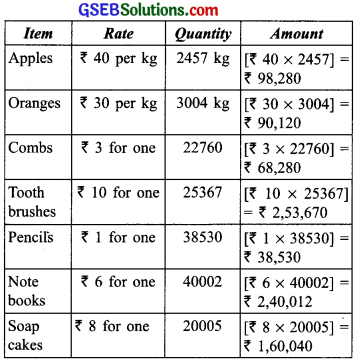Arranging the entries of amount of money received in descending order, we have:
253670; 240012; 160040; 98120; 90120; 68280; 38530
Obviously, the highest amount of money is received against the item ‘tooth brushes”.
This highest amount of money is 2,53,670.Try These (Page 19)

Question 1.
Round these numbers to the nearest tens: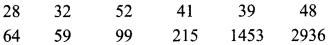Solution:
Number 5 is rounded off to 10.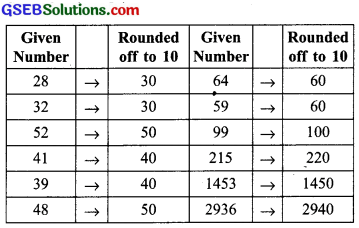Try These (Page 20)

Question 1.
Round off the given numbers to the nearest tens, hundreds and thousands.Solution: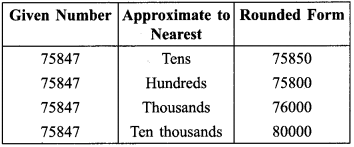Try These (Page 22)

Question 1.
Estimate the following products:
(a) 87 x 313
(b) 9 x 795
(c) 898 x 785
(d) 958 x 387
Solution:
(a) 87 x 313
87 → 90 [Rounding to tens]
313 → 300 [Rounding to hundreds]
Estimated product = 90 x 300 = 27000

(b) 9 x 795
9 → 10 [Rounding to tens]
795 → 800 [Rounding to hundreds]
Estimated product = 10 x 800 = 8000

(c) 898 x 785
898 → 900 [Rounding to hundreds]
785 → 800 [Rounding to hundreds]
Estimated product = 900 x 800 = 720000

(d) 958 x 387
958 → 1000 [Rounding to hundreds]
387 → 400 [Rounding to hundreds]
Estimated product = 1000 x 400 = 400000
Note: Students are requested to make five more such problems and solve themselves.Try These (Page 23)

Question 1.
Write the expressions for each of the following using brackets.
(a) Four multiplied by the sum of nine and two,
(b) Divide the difference of eight en and six by four
(c) Forty – five divided by three times the sum of three and two.
Solution
(a) 4 x (9 + 2)
(b) (18 – 6) ÷ 4
(c) 45 ÷ [3(3 + 2)]

Question 2.
Write three different situations for (5 + 8) x 6. (One such situation is: Sohani and Reeta work for 6 days; Sohani works 5 hours a day and Reea 8 hours a day. How many hours do both of them work in a week?)
Solution:
Situation – I: Rahul pays ₹ 5 for a morning tea and ₹ 8 for the evening coffee daily. He takes tea and coffee for six days in a week. What is his weekly expenses for tea and coffee?

Situation – II: Prema and Shanti work for six days. Prema earns ₹ 5 per day and Shanti earns ₹ 8 per day. What do they earn together in six days?

Situation – III: Prateek read 5 pages of a novel in the morning and 8 pages in the evening. How many pages did Prateek read in 6 days?Question 3.
Write five situations for the following where brackets would be necessary
(a) 7(8 – 3)
(b) (7 + 2)(10 – 3)
Solution:
(a) 7(8 – 3)
Situation – I: Parti is a domestic lady. She charges ₹ 8 per day to clean a house but gives back ₹ 3 to the house owner for saving. What amount does she carry in hand from 7 houses?

Situation – II: What is the seven times the difference of eight and three?

Situation – III: A driver is supposed to work for 8 hours a day. But due to a certain reason, he had to go home 3 hours early daily for 7 days. What number of hours he could attend his duty on these seven days?

Situation – IV: Seven children with ₹ 8 each went to market. Each of them bought pencils costing ₹ 3. What total money is left with them?

Situation – V: There are seven containers having 8 litres of oil each. If 3 litres of oil is taken out from each container, then how many litres of total oil is left in the containers?
(b) (7 + 2)(10 – 3)

Situation – I: A restaurant serves tiffin-lunch for 7 adults and 2 children in each of 10 houses of a society. On a Sunday, 3 houses were locked. How many persons were served lunch on that Sunday?

Situation – II: 7 persons hired a van upto a metro-station, for ₹ 10 per head. The van-driver added 2 more passengers and reduced the fare by ₹ 3 per head, What was the driver’s total collection as fare?

Situation – III: Rahul gets ₹ 10 per day as pocket money. He spends ₹ 3 per day. In December, for one week he is given a bonus-pocket money for 2 days. What is his saving for that week of the month of December?

Situation – IV: A part-time gardener was hired for 7 days a month. He was supposed to work 10 hours daily. In a particular month he worked daily 3 hours less but extended his working for 2 more days. Find the total number of hours for which he worked in that month.

Situation – V: A team of 7 sales girls and 2 managers work for 10 hours daily in a garment shop. On Saturday, the shop was closed 3 hours cariy. How many total number of hours did the team work in the shop for the day?Try These (Page 25)

Question 1.
Write in Roman Numerals:
1. 73
2. 92
Solution:
1. 73 = LXXIII
2. 92 = XCII